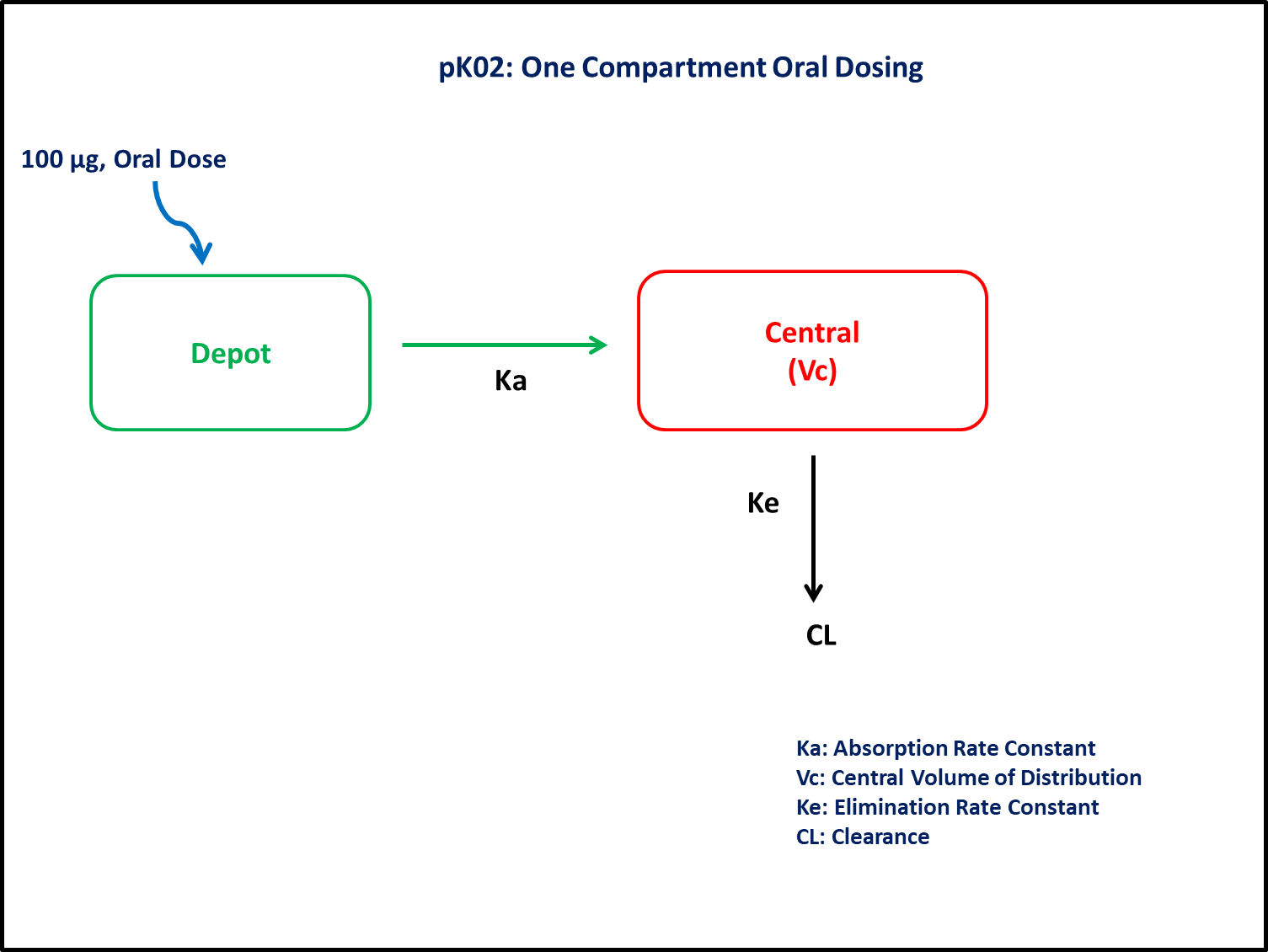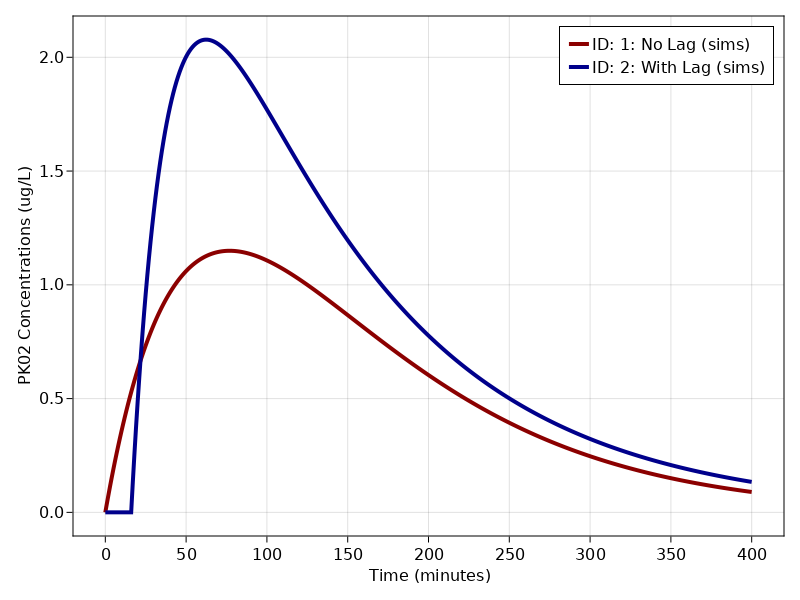# Exercise PK2 - One Compartment Oral Dosing

### Background

• Structural model - 1 compartment with first order absorption (without/with Lag time)

• Route of administration - Oral

• Dosage Regimen - 100 μg Oral

• Number of Subjects - 1### Learning Outcome

By the application of the present model, we will learn how to simulate model for first order input model with and without lag-time.

### Objectives

In this exercise you will learn how to

• Simulate an Oral One Compartment (without/ with lag-time). Assuming oral bioavailability of 100%. The interpretation V includes bioavailability (i.e., it is really estimating V/F).

• Write a differential equation for a one-compartment model

### Libraries

call the "necessary" libraries to get start.

using Random
using Pumas
using PumasUtilities
using CairoMakie


### Model

In this one compartment model, we administer dose in Depot compartment at time= 0.

pk_02        = @model begin
desc = "One Compartment Model with lag time"
timeu = u"minute"
end

@param begin
"Absorption rate constant (1/min)"
tvka     ∈ RealDomain(lower=0)
"Elimination rate constant (1/min)"
tvkel    ∈ RealDomain(lower=0)
"Volume (L)"
tvvc     ∈ RealDomain(lower=0)
"Lag Time (mins)"
tvlag    ∈ RealDomain(lower=0)
Ω        ∈ PDiagDomain(4)
"Proportional RUV"
σ²_prop  ∈ RealDomain(lower=0)
end

@random begin
η        ~ MvNormal(Ω)
end

@pre begin
Ka       = tvka * exp(η)
Kel      = tvkel * exp(η)
Vc       = tvvc * exp(η)
end

@dosecontrol begin
lags     = (Depot=tvlag * exp(η),)
end

@dynamics begin
Depot'   = -Ka*Depot
Central' =  Ka*Depot - Kel*Central
end

@derived begin
"""
PK02 Concentration (ug/L)
"""
cp       = @. Central/Vc
"""
PK02 Concentration (ug/L)
"""
dv       ~ @. Normal(cp, sqrt(cp^2*σ²_prop))
end
end

PumasModel
Parameters: tvka, tvkel, tvvc, tvlag, Ω, σ²_prop
Random effects: η
Covariates:
Dynamical variables: Depot, Central
Derived: cp, dv
Observed: cp, dv


### Parameters

The compound follows a one compartment model, in which the various parameters are as mentioned below:

• $Ka$ - Absorption Rate Constant (min⁻¹)

• $Kel$ - Elimination Rate Constant(min⁻¹)

• $Vc$ - Central Volume of distribution (L)

• $tlag$ - Lag-time (min)

• $Ω$ - Between Subject Variability

• $σ$ - Residual error

param = [
(tvka    = 0.013,
tvkel   = 0.013,
tvvc    = 32,
tvlag   = 0,
Ω       = Diagonal([0.0,0.0,0.0,0.0]),
σ²_prop = 0.015),

(tvka    = 0.043,
tvkel   = 0.0088,
tvvc    = 32,
tvlag   = 16,
Ω       = Diagonal([0.0,0.0,0.0,0.0]),
σ²_prop = 0.015)
]


### Dosage Regimen

In this section the Dosage regimen is mentioned:

• Oral dosing of 100 μg at time=0 for a single subject

ev1  = DosageRegimen(100, time = 0, cmt = 1)
pop  = map(i -> Subject(id = i, events = ev1), ["1: No Lag", "2: With Lag"])

Population
Subjects: 2
Observations:


### Simulation

When simulating a single subject, we can pass in an array of parameters to visualize multiple curves for the same subject.

Random.seed!(123)
sim = map(zip(pop, param)) do (subj, p)
return simobs(pk_02, subj, p, obstimes = 0:1:400)
end

Simulated population (Vector{<:Subject})
Simulated subjects: 2
Simulated variables: cp, dv


### Visualize results

From the plot below, we can clearly identify the subject with and without lag time.

f, a, p = sim_plot(pk_02, sim,
observations = :cp,
color = :redsblues, linewidth = 4,
axis = (xlabel = "Time (minutes)",
ylabel = "PK02 Concentrations (ug/L)",
xticks = 0:50:400,))
axislegend(a)
f